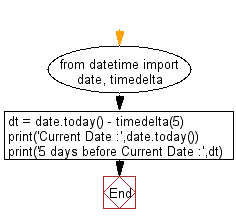﻿ Python: Subtract five days from current date - w3resource

# Python: Subtract five days from current date

## Python datetime: Exercise-5 with Solution

Write a Python program to subtract five days from the current date.

Sample Solution:

Python Code:

``````from datetime import date, timedelta
dt = date.today() - timedelta(5)
print('Current Date :',date.today())
print('5 days before Current Date :',dt)
```
```

Sample Output:

```Current Date : 2017-05-05
5 days before Current Date : 2017-04-30
```

Flowchart:Python Code Editor: